# Exploring NHL Stanley Cup Champion's Points Percentage In Four GGPlots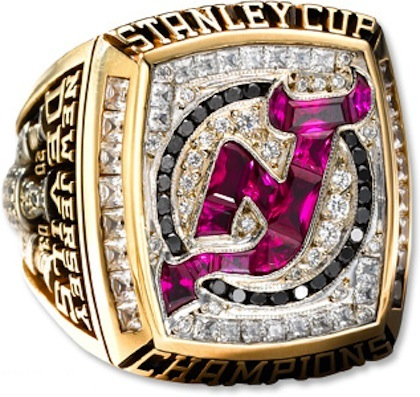## Motivation

While browsing Reddit’s r/DataIsBeautiful sub-reddit I came across a post from Fabio Votta showing a beeswarm plot of US County vote share in the 2020 Election. Having never seen a beeswarm plot before I wanted to come up with an excuse to try it out. As an NHL fan, I decided to look at the Points Percentage of NHL Stanley Cup champions. This analysis will use information from hockey-reference.com and `ggplot` to visualize the information.

## Getting the Data

The data for this analysis will come from hockey-reference.com which provides statistics on the Stanley Cup Champion teams from 1918 through 2020 (with some exceptions). The points percentage is provided as a direct column in the table.

### Setting Up Libraries

The libraries used in this analysis include stalwarts like tidyverse as well as ggplot extensions such as `ggtext`, `ggbeeswarm`, `ggridges`, `ggimage` to do different visualizations. The `extrafont` package enables the use of the fonts installed on my machine in ggplots. The `loadfonts(device = "win")` function loads the additional fonts (if running for the first time the `font_import()` function needs to be called to build the references).

``````library(tidyverse) # Data Manipulation and Visualizations
library(rvest) # Web Scraping the NHL Champion Data & Team Colors
library(ggbeeswarm) # Creating Beeswarm Plots
library(ggtext) # Enabling Use of Markdown in ggplots
library(ggridges) # Creating Ridge Density Plots
library(ggimage) # Creating Plots with Images as the Points
library(glue) # Package for String Manipulation
library(extrafont) # Package to enable use of additional fonts for plotting

### Getting the Data on the Champions

The points data for the Stanley Cup Champions comes from hockey-reference.com. I’ll scrape the table from this website by using `rvest` and referencing the CSS class `.stats_table`. Since there’s only one table on the page I can use `html_node` vs. `html_nodes`. Eventually I’m planning on joining some additional data to this data frame so I’m doing a minimal amount of data cleaning such as changing the Chicago Blackhawks to 1 word so that it matches the second data set. Additionally I’m renaming the points percentage column to something more R friendly.

``````nhl_data <- read_html('https://www.hockey-reference.com/awards/stanley.html') %>%
html_node(css = '.stats_table') %>%
html_table() %>%
mutate(Team = str_replace_all(Team, "Black Hawks", "Blackhawks")) %>%
rename(pts_pct = `PTS%`)``````

### Getting Data on Team Colors

For one of the future plots I want to use each team’s color to represent their data. This information comes from teamcolorcodes.com. Each team page has a formulaic URL where the team name is ‘-’ delimited. Since this page only has information on current teams, older teams like the Toronto Arenas or Montreal Maroons will not appear. Typically, these names might wind up breaking a loop when they throw an error. However, the use of the `possibly()` function from `purrr` will accommodate the error handling. The `possibly()` function wraps another function and has an `otherwise` parameter that allows the user to say what the function should provide in case of an error.

In this case, the `possibly()` function wraps an anonymous function that:

• Takes a string for a team name, `t`, which is converted to lower-case and has the spaces replaced with dashes
• Scrapes the first instance of the `.colorblock` CSS class from the teamcolorcodes.com webpage for the specific team as text.
• Performance a regular expression map for the HEX code for the color
• Since `str_match` returns a list where the first element is the entire match and each additional element represents a capture group, pulls the 2nd element from the list.
• Finally, the function returns a 1-row tibble with the team name, `t`, and the HEX code, named `color.`
• In the case that there’s an error, the function will return a 1-row tibble with the team value set to ‘non-match’ and the color value set to `NA`.

The `map_dfr` function from `purrr` is used to run the above function for all unique team names and appends the results into a data.frame.

``````get_color <-  possibly(
function(t){
tibble(
team = t,
color = glue("https://teamcolorcodes.com/{t}-color-codes/",
t = str_replace_all(
str_to_lower(t), ' ', '-')
) %>%
html_node(css = ".colorblock") %>%
html_text() %>%
str_match("Hex Color: (#[0-9A-Za-z]{6})") %>%
.[, 2]
)
},
otherwise = tibble(team = "non-match", color = NA_character_))

nhl_colors <- map_dfr(unique(nhl_data\$Team), get_color)``````

### Combining the Data

Finally, the color data is joined to the Champions data. In the cases where there were not matches in the color data, I’m using black as a default color.

``````nhl_w_color <- nhl_data %>%
left_join(nhl_colors, by = c("Team" = "team")) %>%
mutate(
color = if_else(is.na(color), "black", color)
) ``````

## Visualizations

### The Overall Distribution of Points Percentage for NHL Stanley Cup Champions

This code block is a doozy as I did a lot of annotations to add error bars, text labels, arrows, and theme formatting to change what at its heart is a standard density plot.

``````nhl_w_color %>%
ggplot(aes(x = pts_pct)) +
geom_density(fill = '#8394A1') +
annotate("errorbarh",
xmin = quantile(nhl_w_color\$pts_pct, .10),
xmax = quantile(nhl_w_color\$pts_pct, .90),
y = 6,
color = "#e6e7eb") +
annotate("linerange",
x = median(nhl_w_color\$pts_pct),
ymin = 0,
ymax = 5,
color = "#e6e7eb",
lty = 2
) +
annotate("text",
label = "Middle 80% and Median",
y = 6.45,
x = median(nhl_w_color\$pts_pct),
color = "#e6e7eb") +
annotate("text",
label = quantile(nhl_w_color\$pts_pct, .10) %>%
scales::percent(accuracy = 1),
y = 5.2,
x = quantile(nhl_w_color\$pts_pct, .10),
color = "#e6e7eb") +
annotate("text",
label = quantile(nhl_w_color\$pts_pct, .90) %>%
scales::percent(accuracy = 1),
y = 5.2,
x = quantile(nhl_w_color\$pts_pct, .90),
color = "#e6e7eb") +
geom_curve(
x = median(nhl_w_color\$pts_pct),
xend = median(nhl_w_color\$pts_pct)-.005,
y = 6,
yend = 3,
color = "#e6e7eb",
arrow = arrow(length = unit(0.03, "npc")),
size = 1
) +
annotate("text", x = median(nhl_w_color\$pts_pct)-.02, y = 3.3,
label = median(nhl_w_color\$pts_pct) %>%
scales::percent(accuracy = 1),
color =  "#e6e7eb") +
labs(title = "Points Percentage of Stanley Cup Champions (1918 - 2020)",
caption = "*Source: hockey-reference.com*",
x = "Points %",
y = ""
) +
scale_x_continuous(labels = scales::percent_format(accuracy = 1)) +

cowplot::theme_cowplot() +
theme(
text = element_text(color = "#e6e7eb", family = 'BentonSans Regular'),
plot.background = element_rect(fill = "#1a1c2e"),
axis.text = element_text(color = "#e6e7eb"),
axis.ticks = element_line(color = "#e6e7eb"),
axis.line = element_line(color = "#878890"),
plot.caption = element_markdown(),
axis.title.y = element_blank(),
axis.text.y = element_blank(),
axis.ticks.y = element_blank(),
axis.line.y = element_blank(),
plot.title = element_text(hjust = .5)
)``````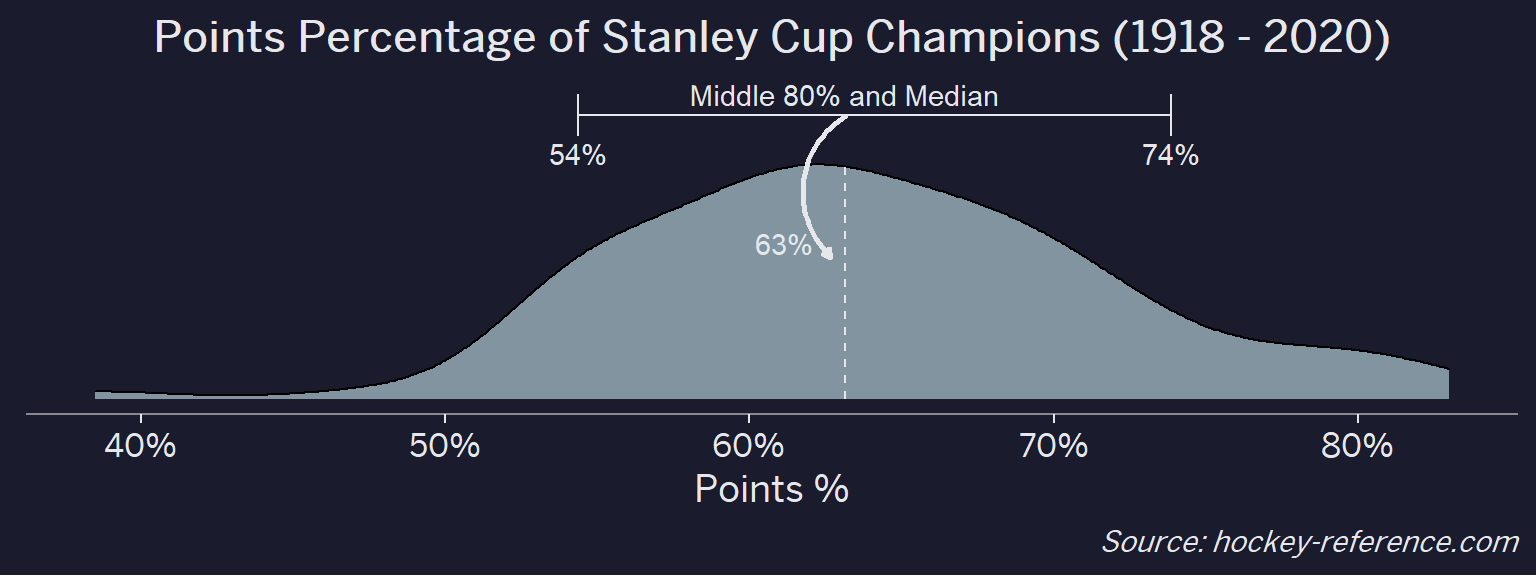Of the 100 champions that there is data for, the median points percentage is 63% while the middle 80% spans 54% - 74%. Ultimately this makes sense since you’d expect a champion to do better than just 50%. However, there are some teams that are really great and have >80% points percentages and a few instances of unlikely champions with a points percentage in the 40s.

### Has the Distribution of Champion’s Points Percentages Changed By Decade

To see the density curves over time one approach would be to facet by decade and show each decade in its own panel. Another approach is to use the `ggridges` package to make a ridge density plot to have each density curve on its own line. The package is very easy to use as its primarily adding a `y` value and then using `geom_density_ridges` vs. `geom_density`.

### Looking the Points Percentage for Each Team

At the beginning of the post I mentioned that seeing a beeswarm plot provided the motivation for this post. Now I’ll actually create it. The following plot will have one point for each champion which will be highlighted by the team’s colors when that team’s tab is selected.

The two things to note in this code block is:

• The tabset is dynamically generated by the markdown by setting the chunk setting to `results='asis'` and then using `cat()` to add the HTML for the tabs through a for-loop.
• In vanilla RMarkdown, the tabset effect is really easy to do with `{.tabset}` but in Blogdown/Hugo its a bit trickier to nail the formatting. But its doable by referencing the bootstrap.js documentation To make things look decent, I’m omitting the code chunk but will include it at the bottom.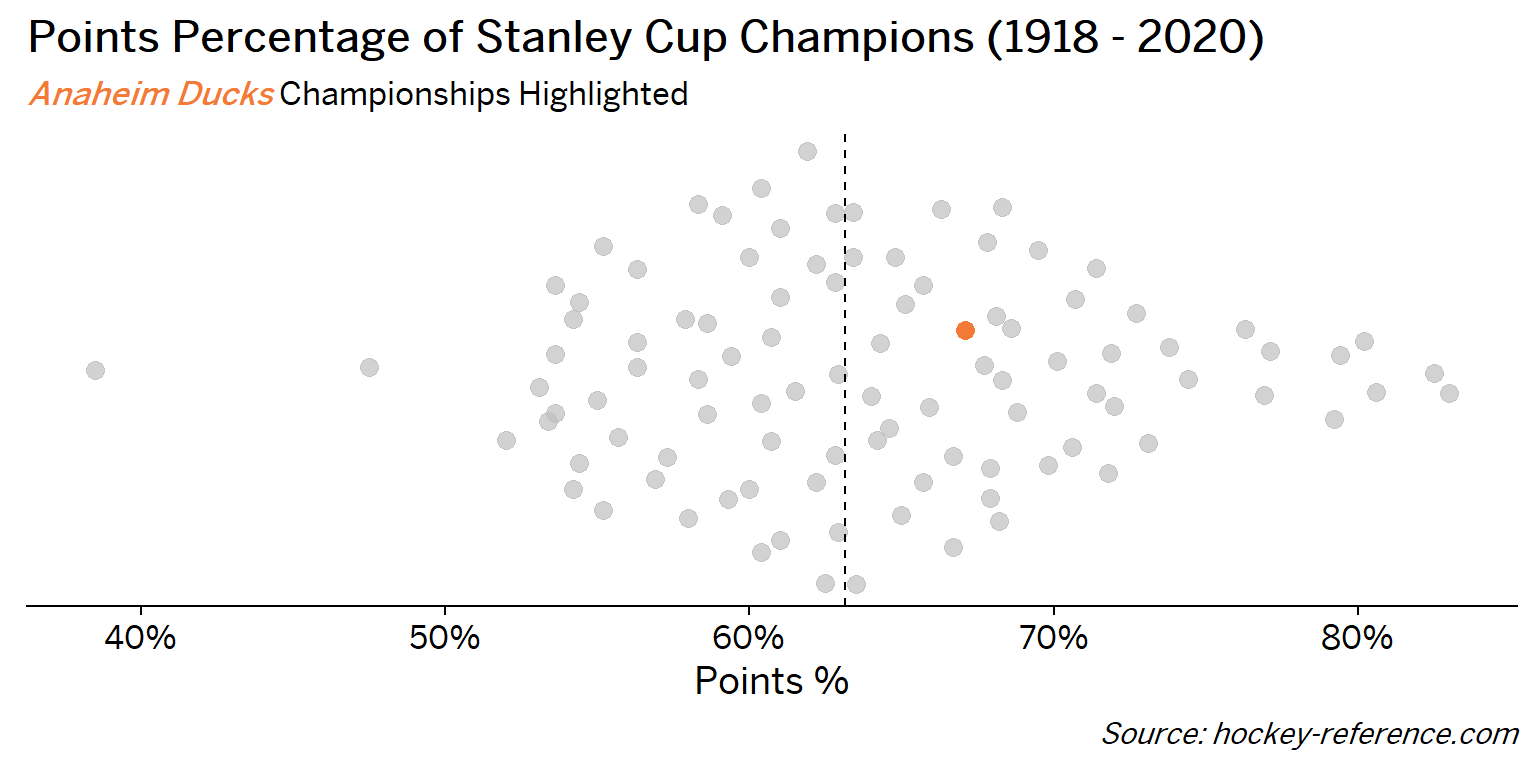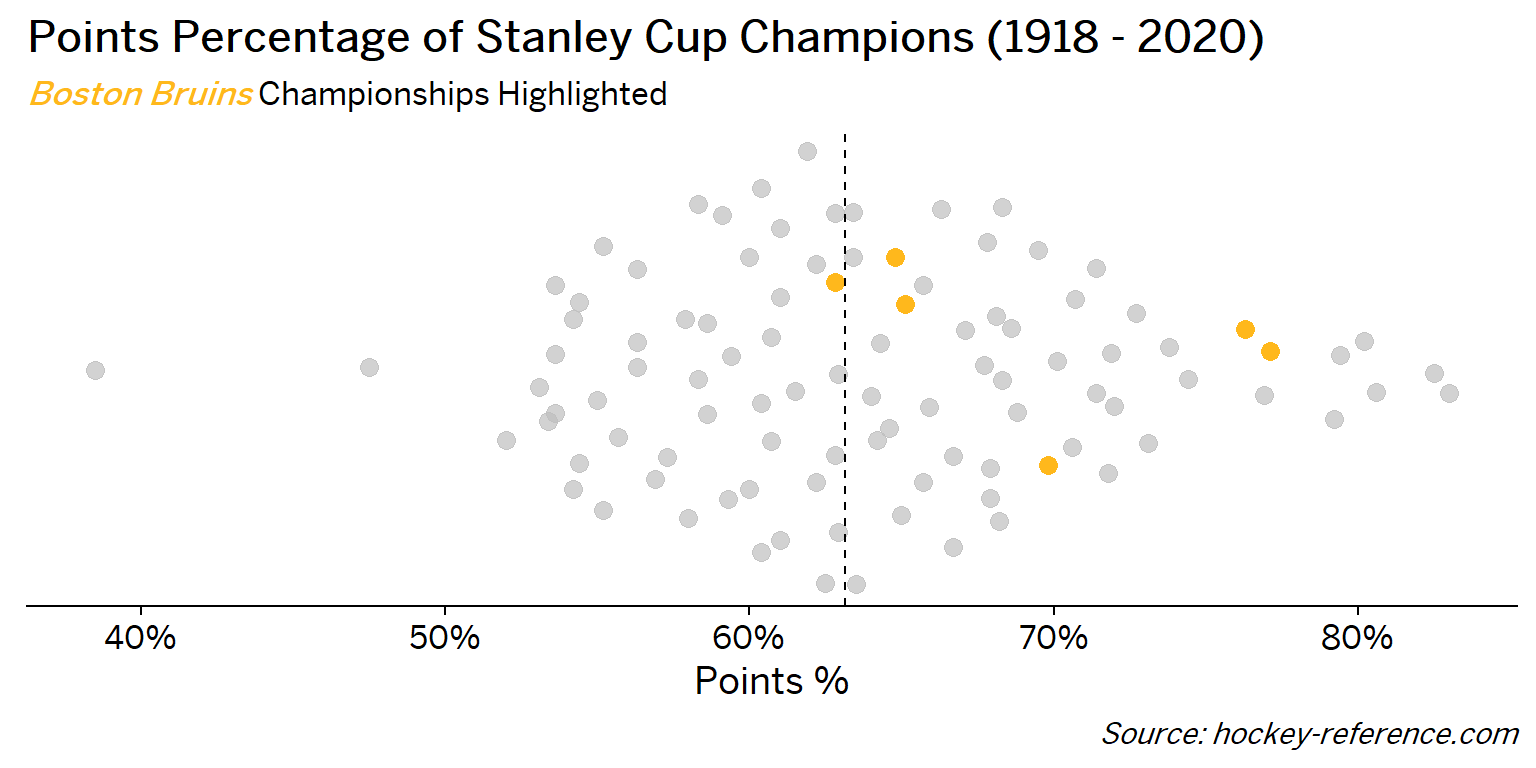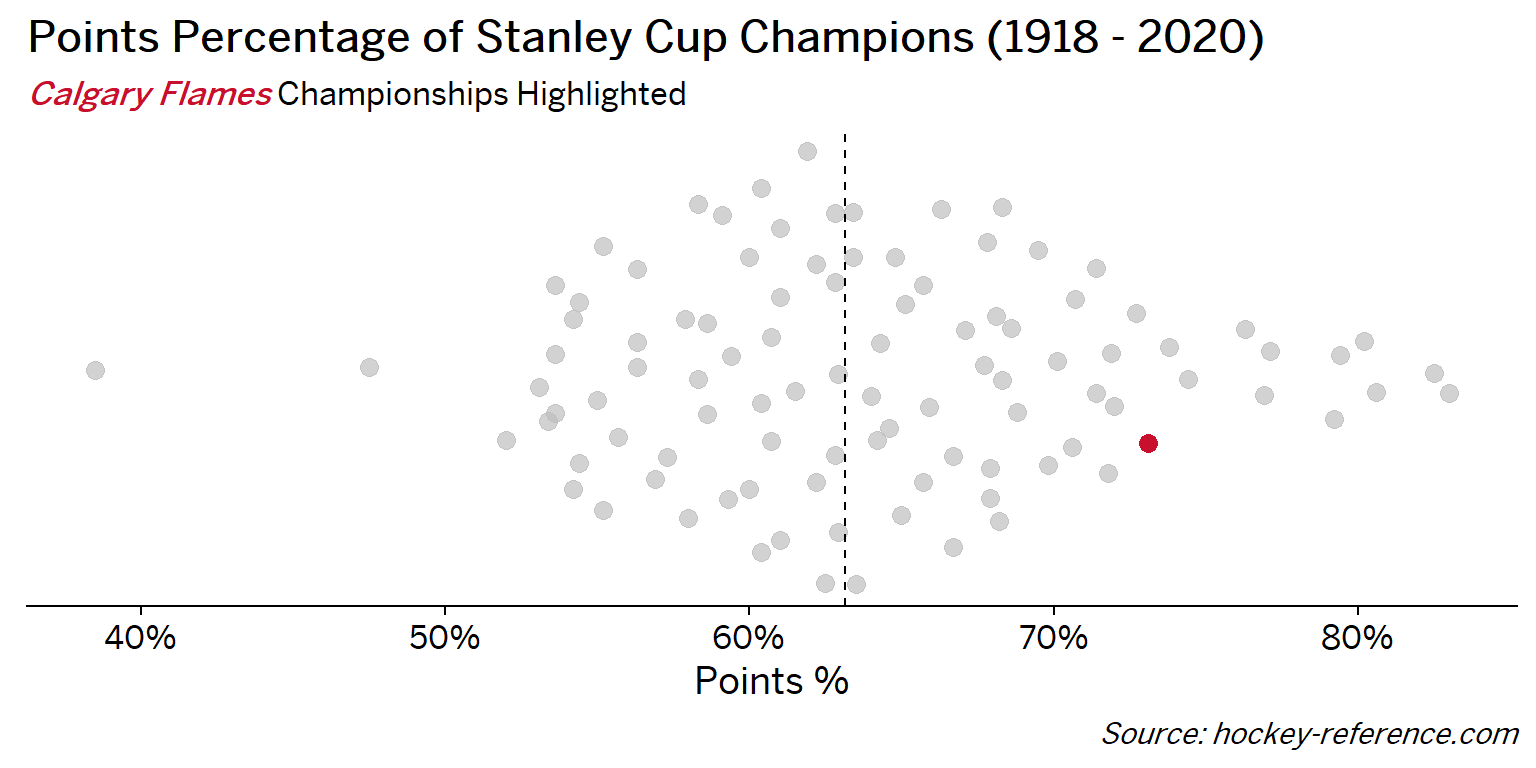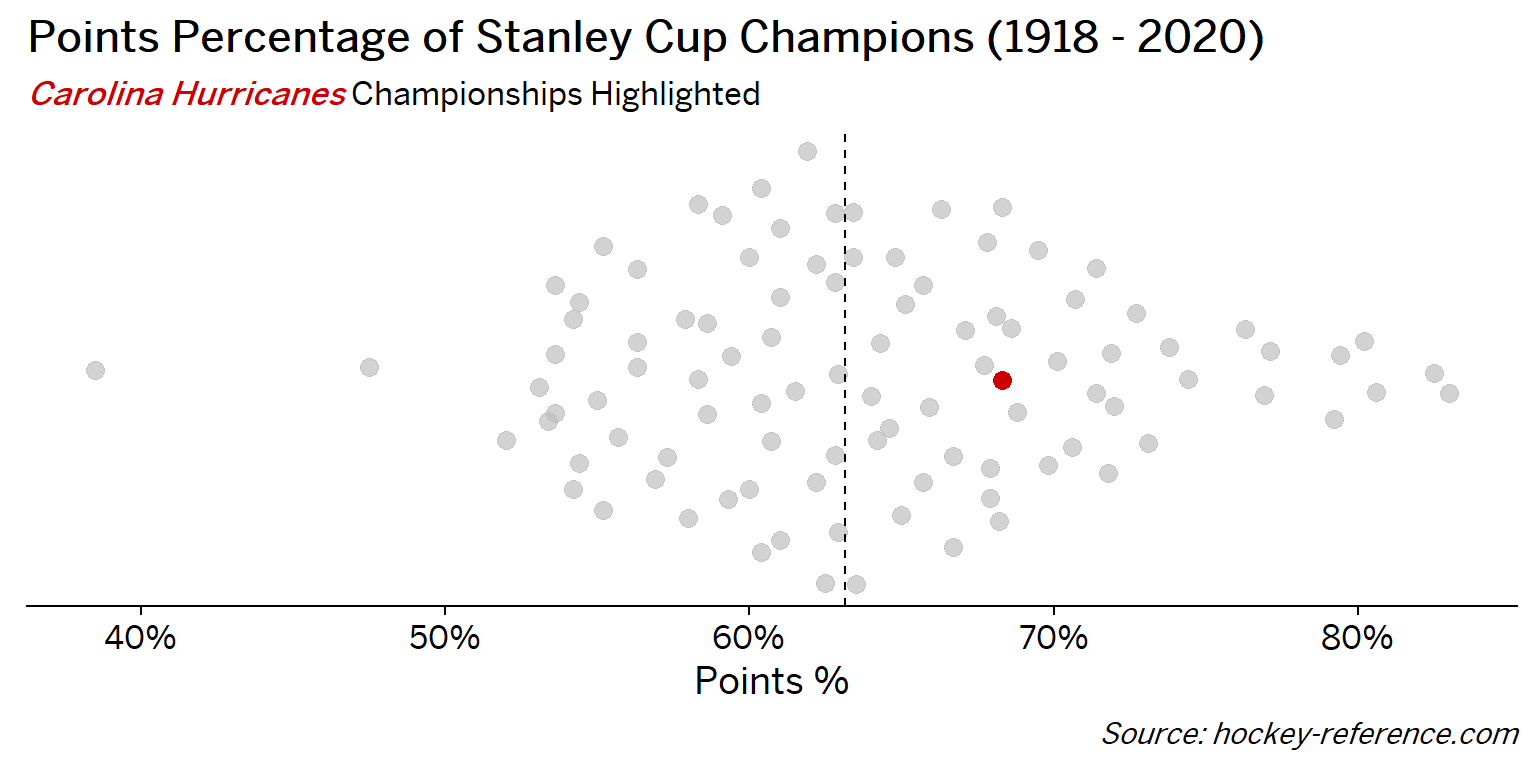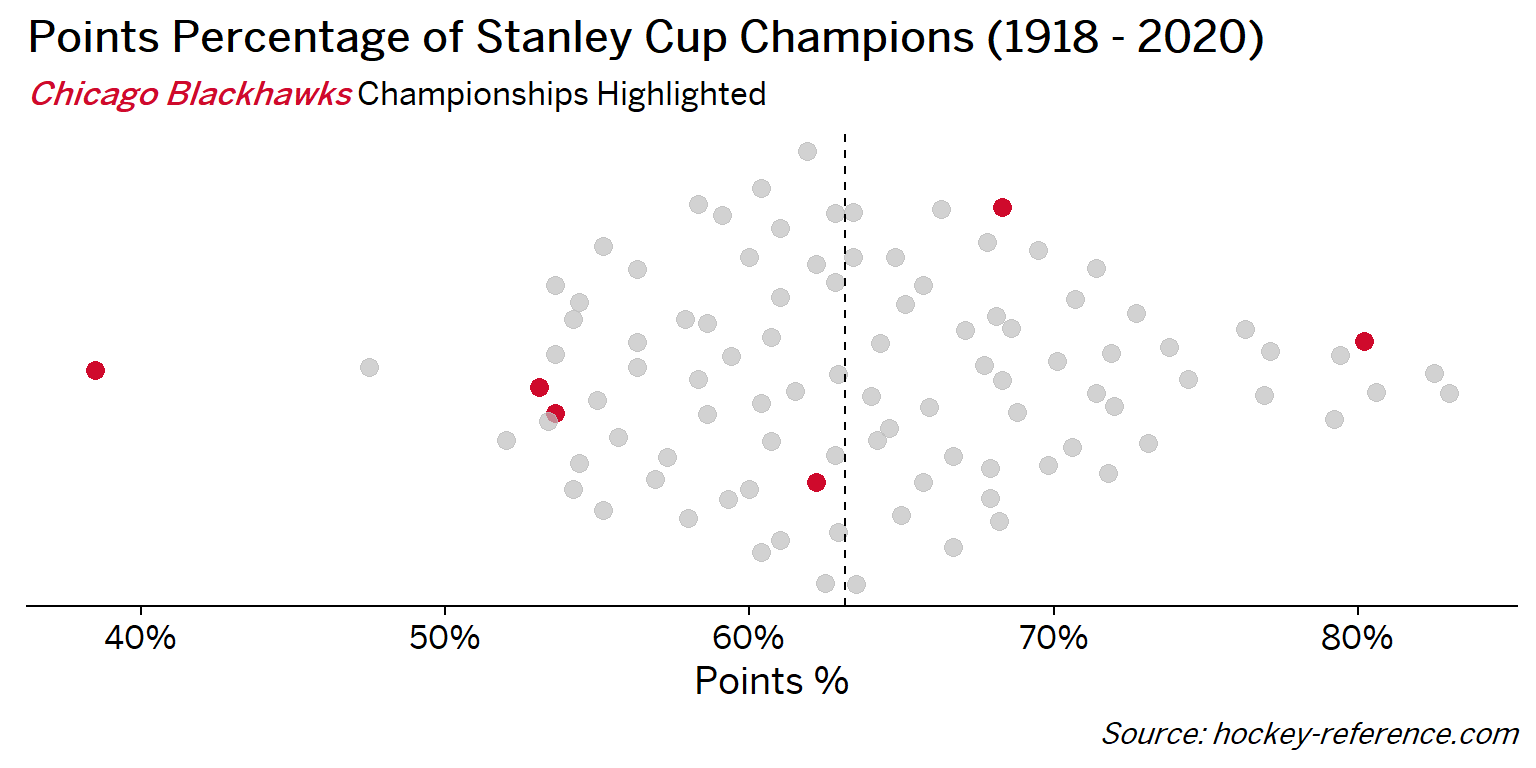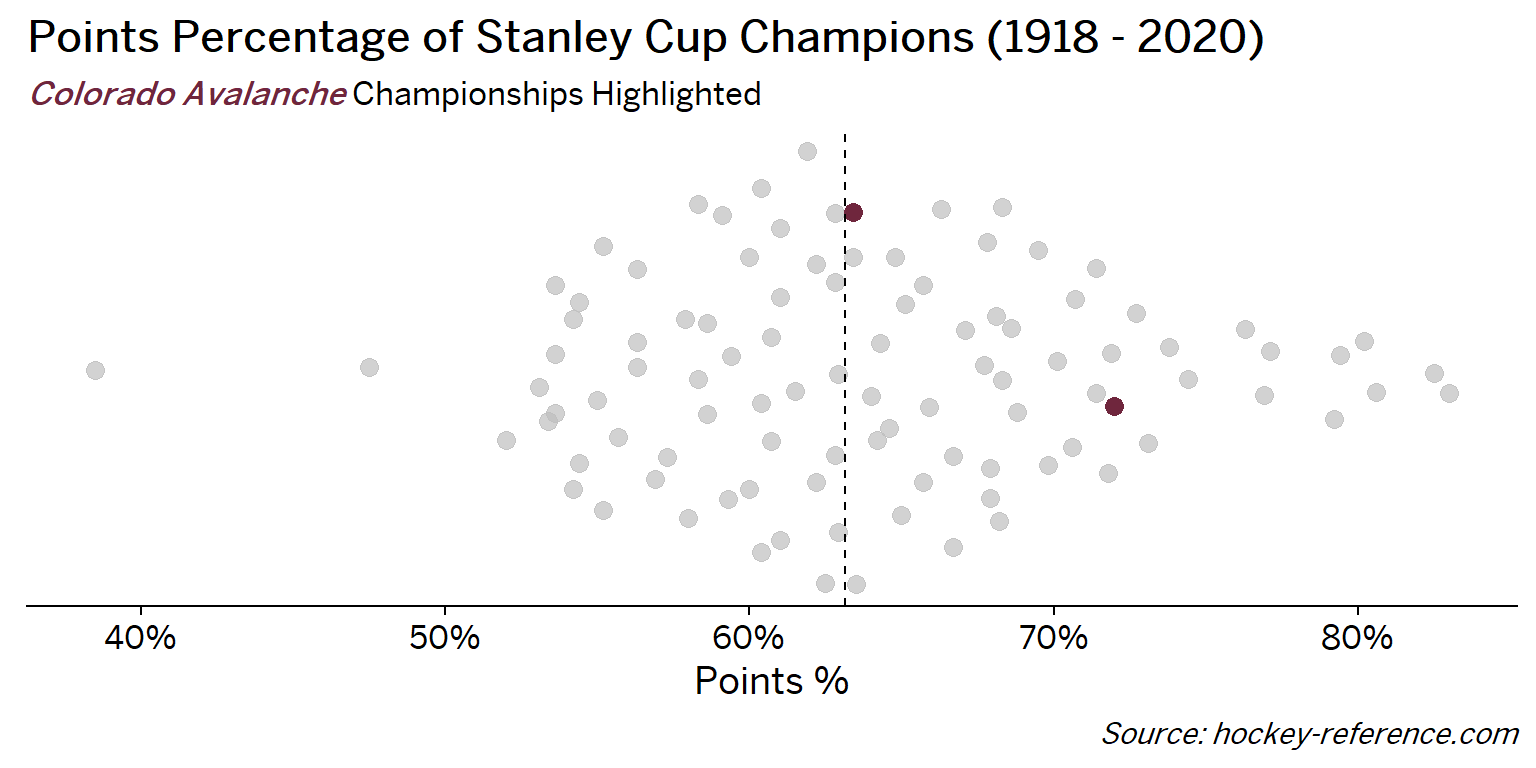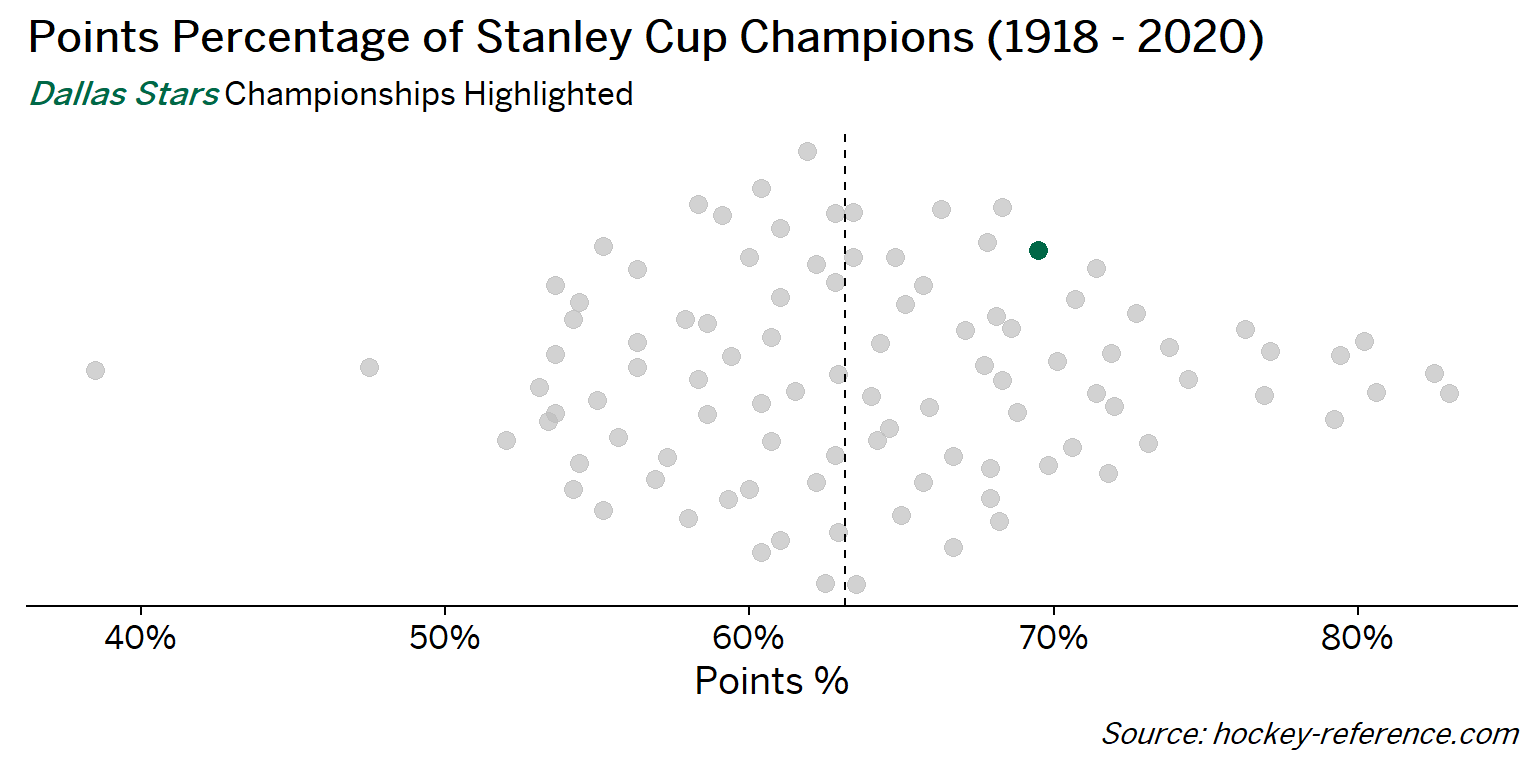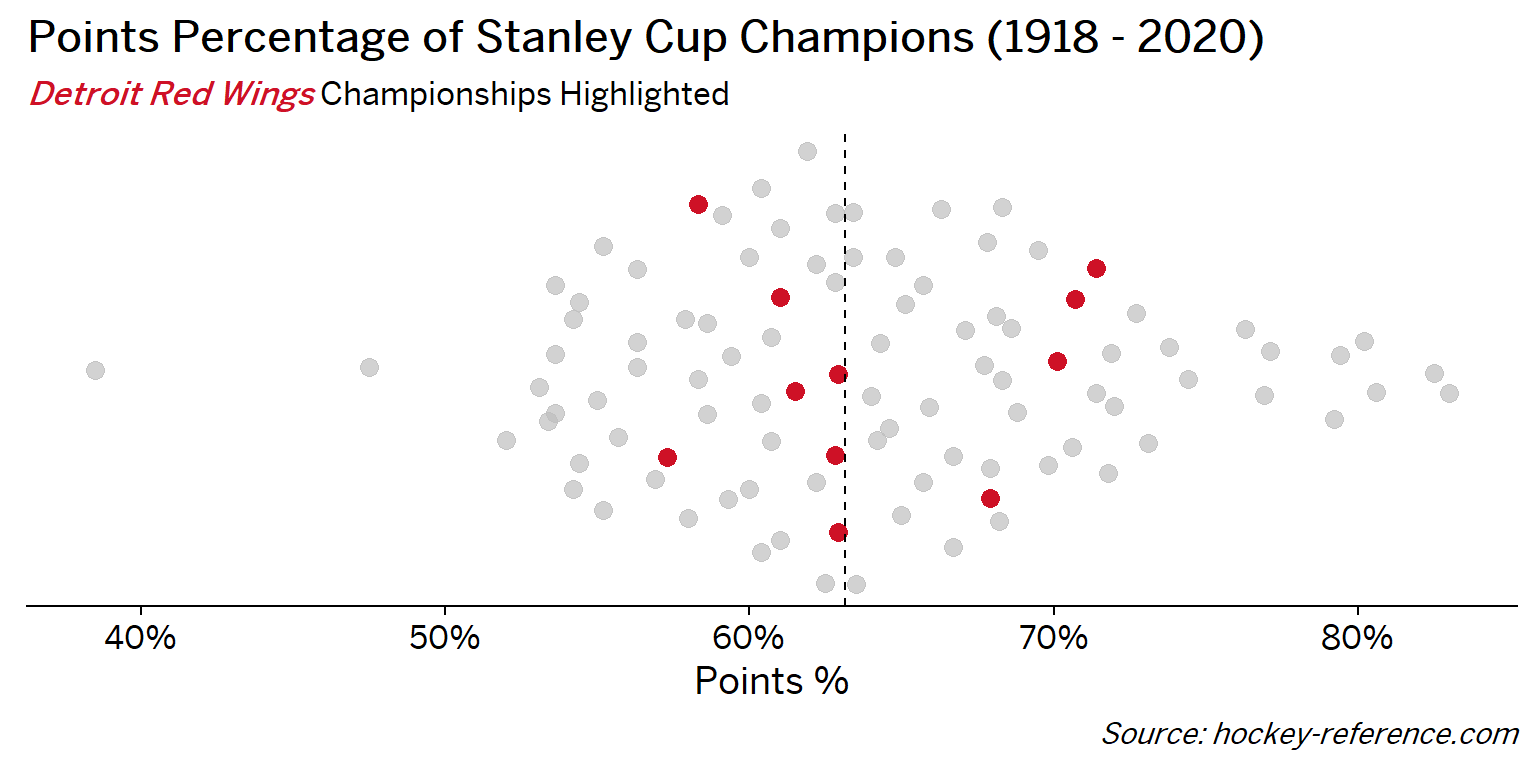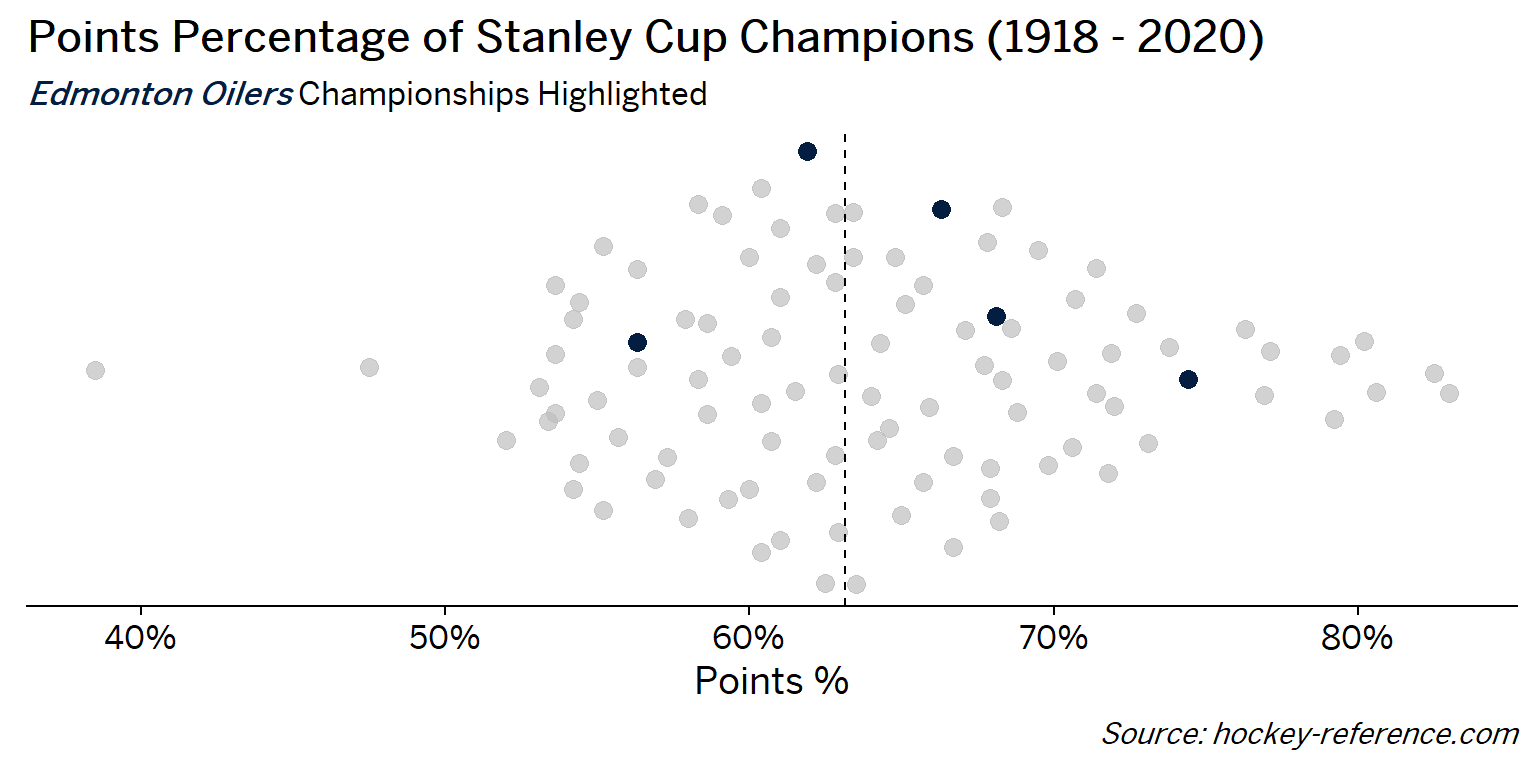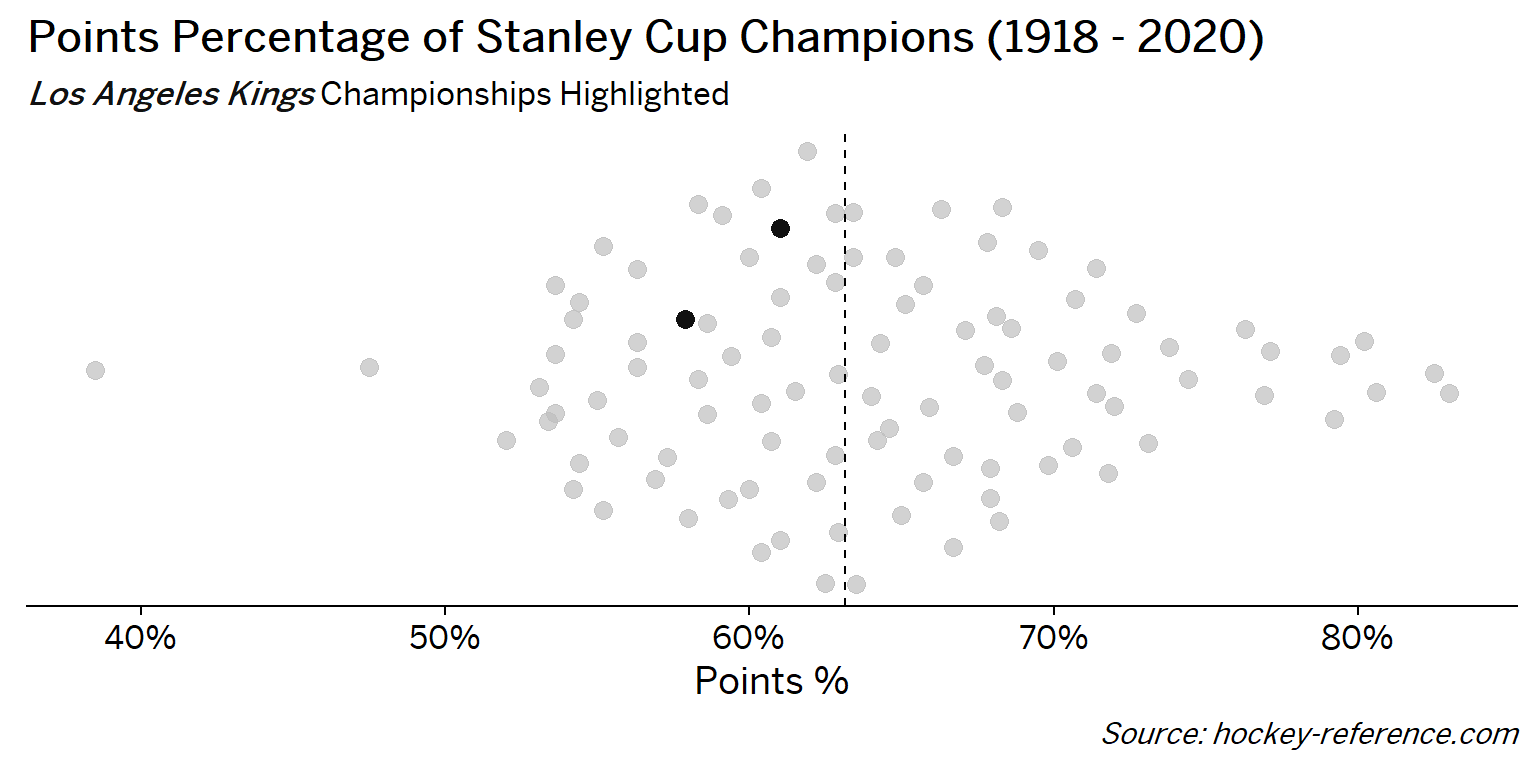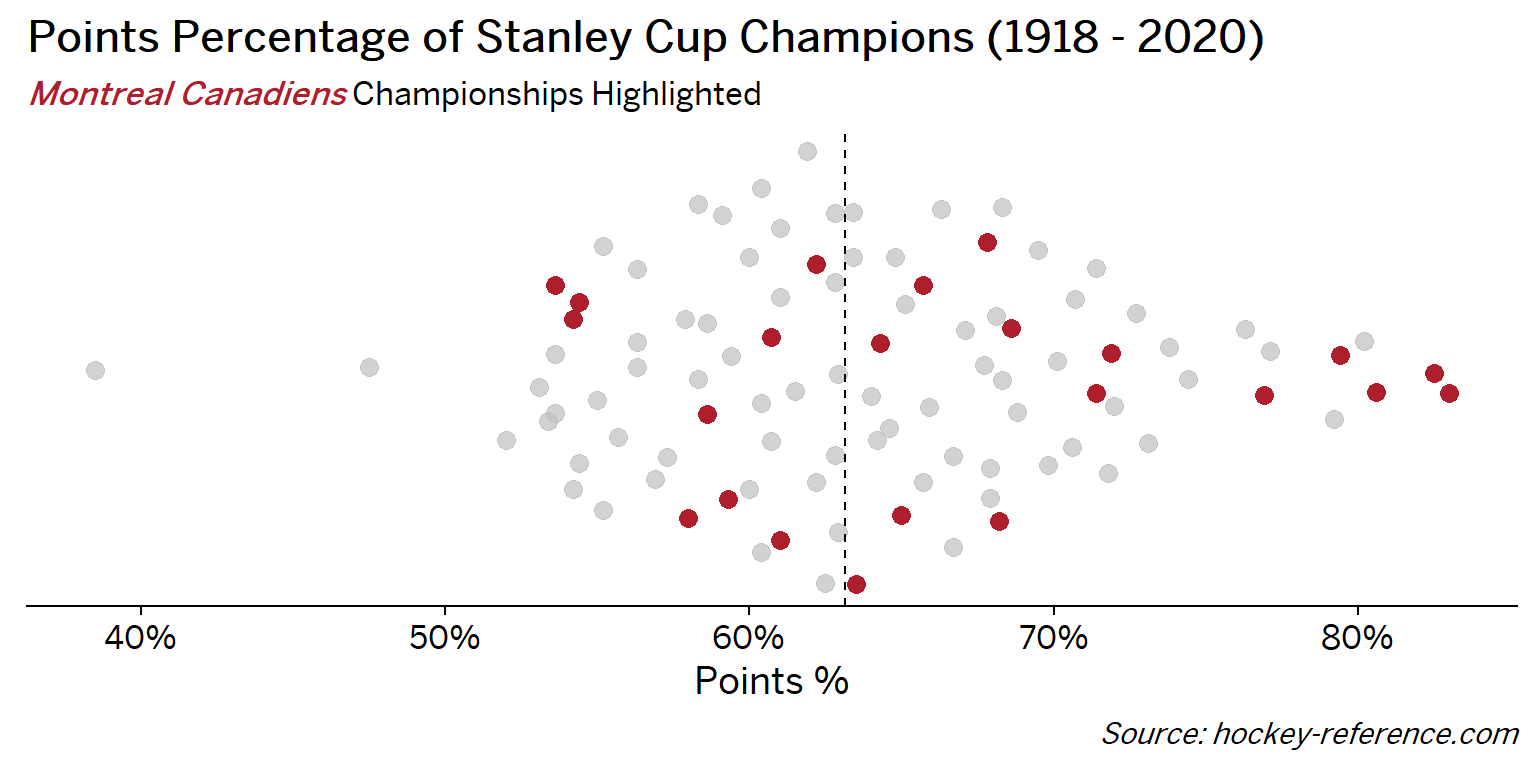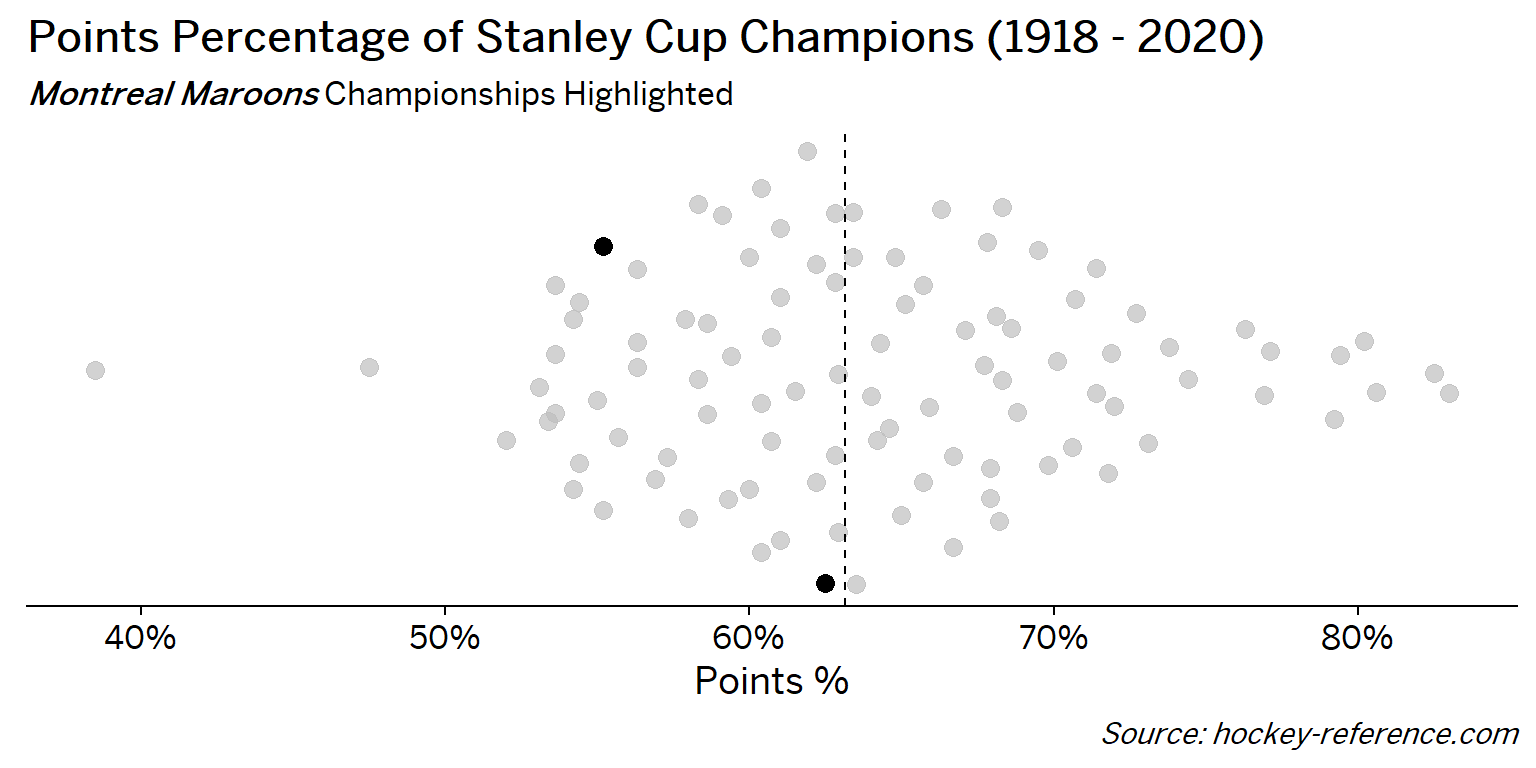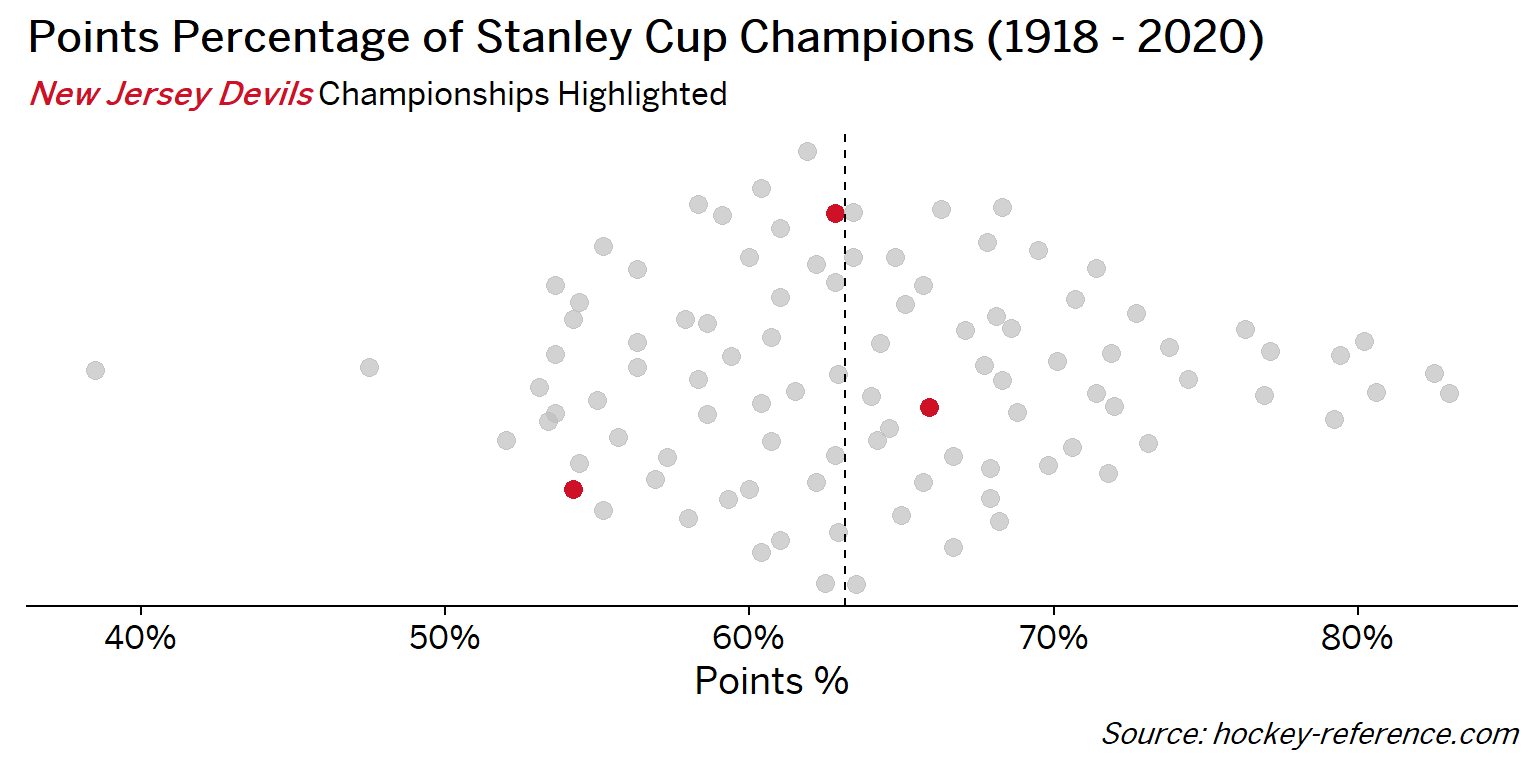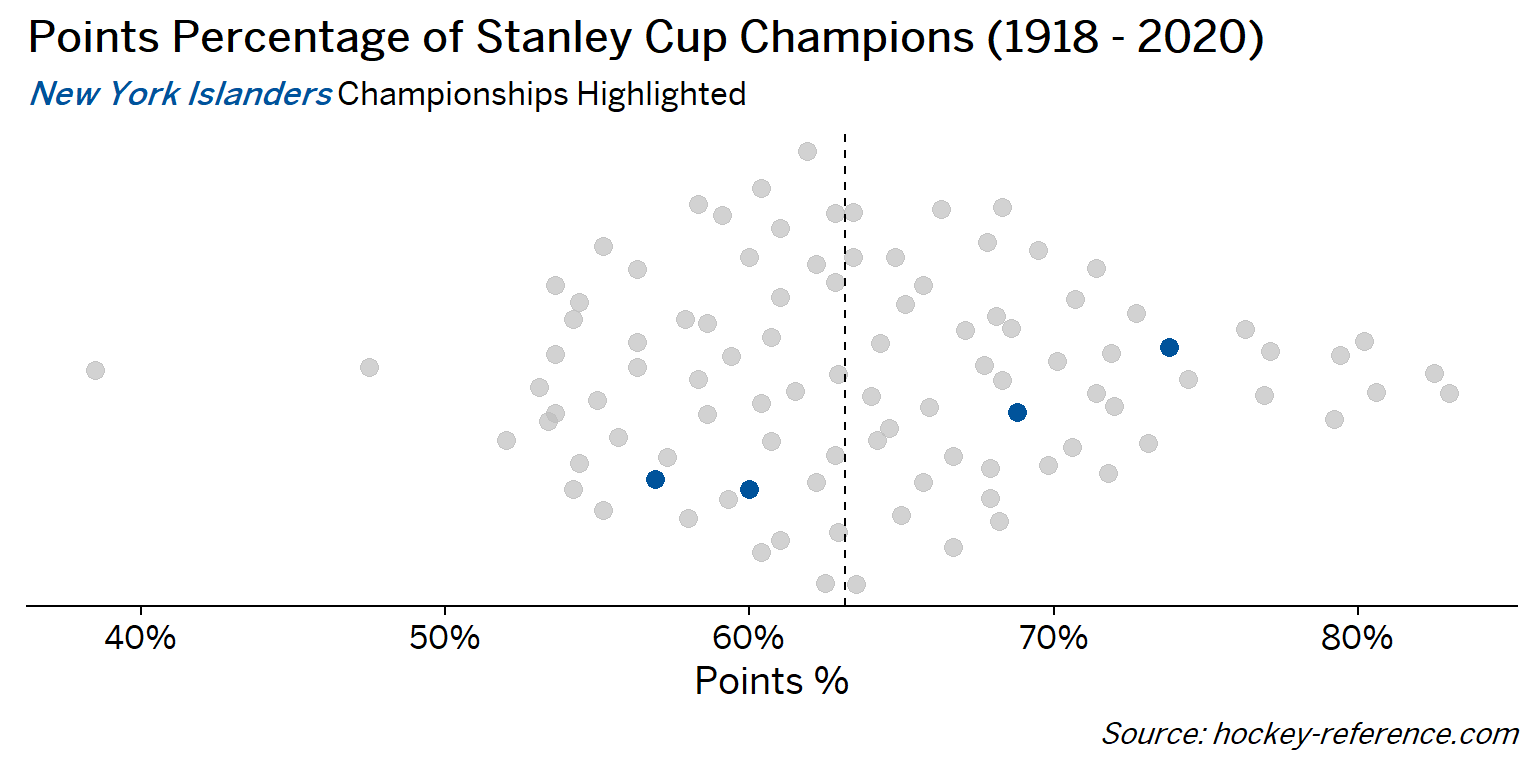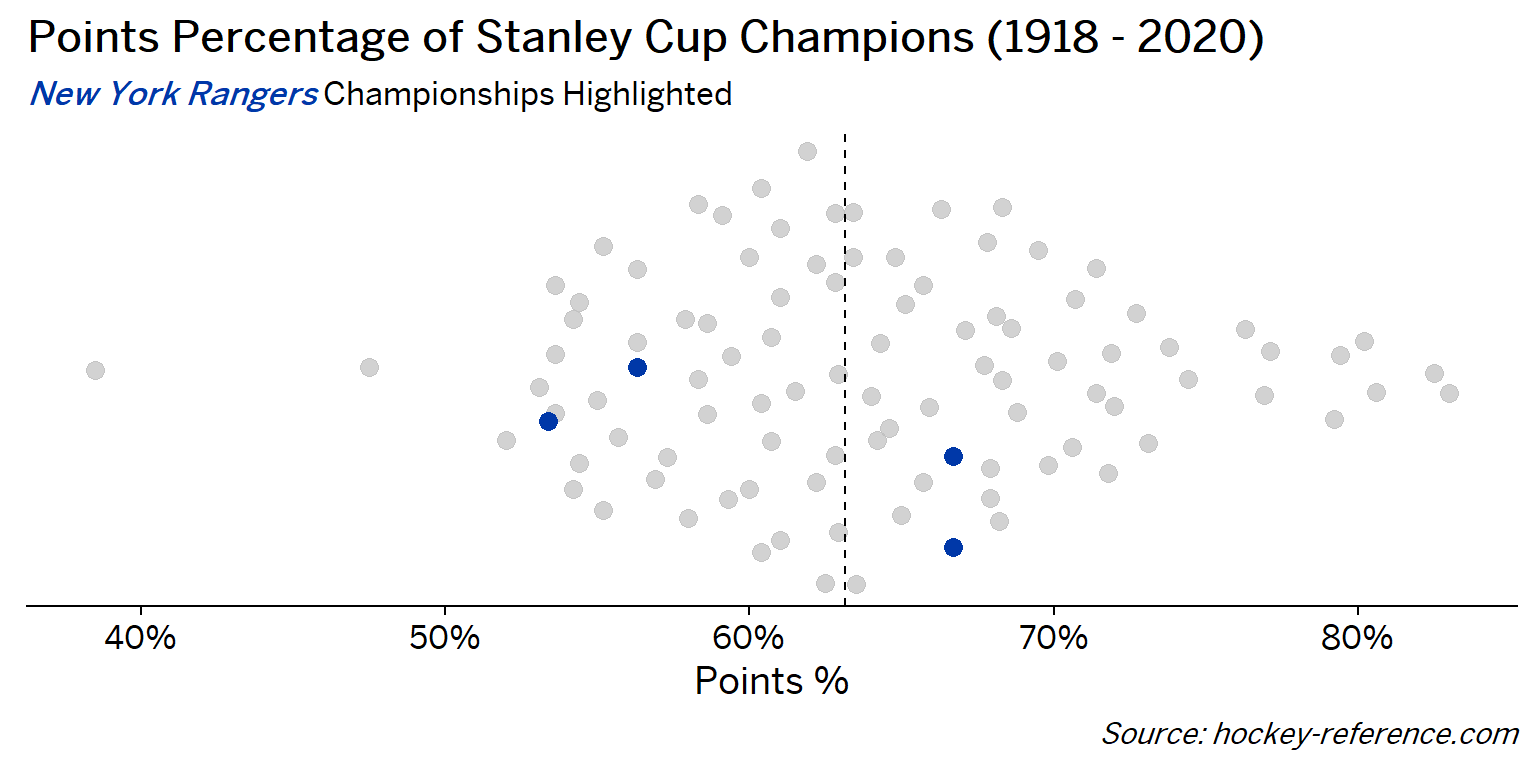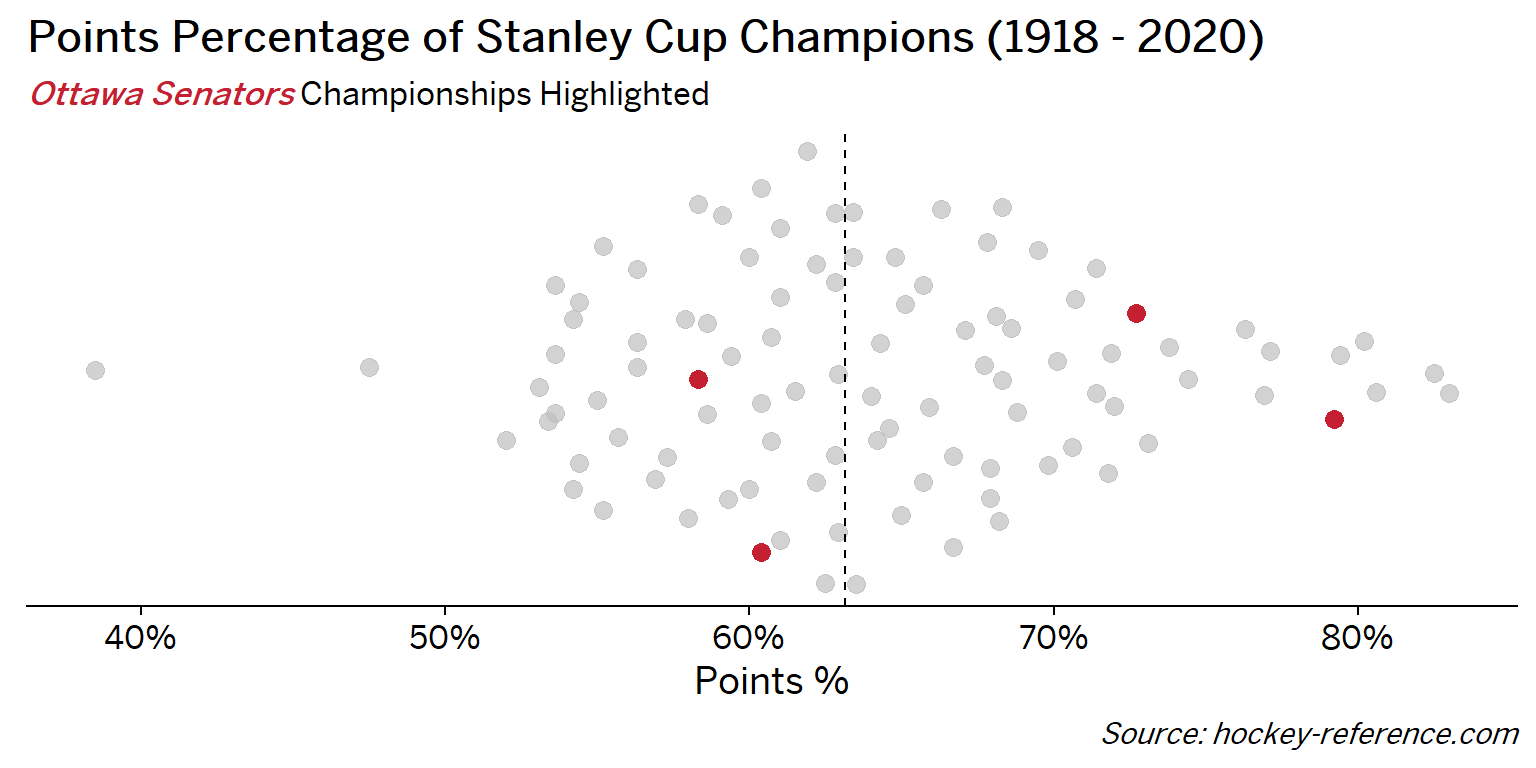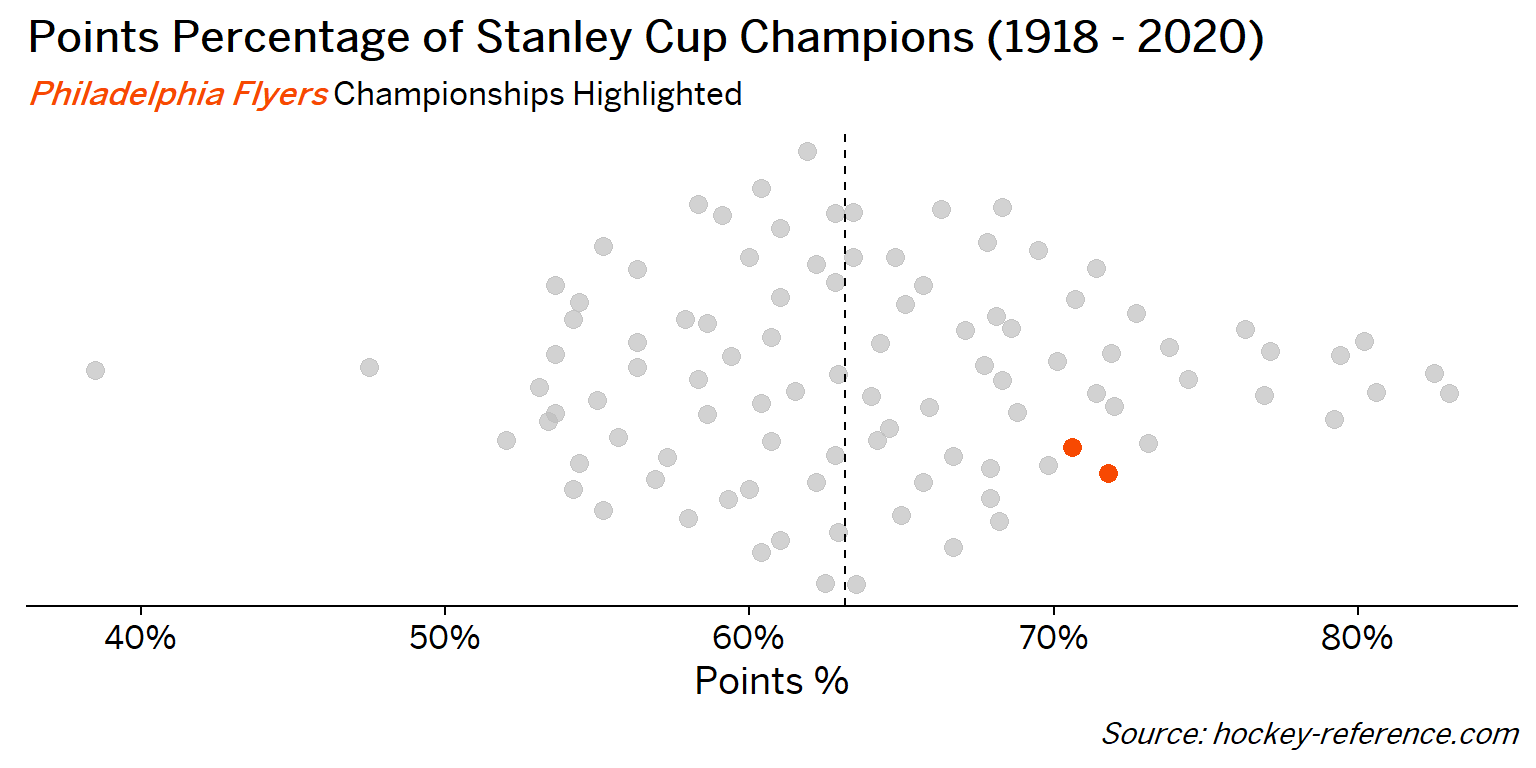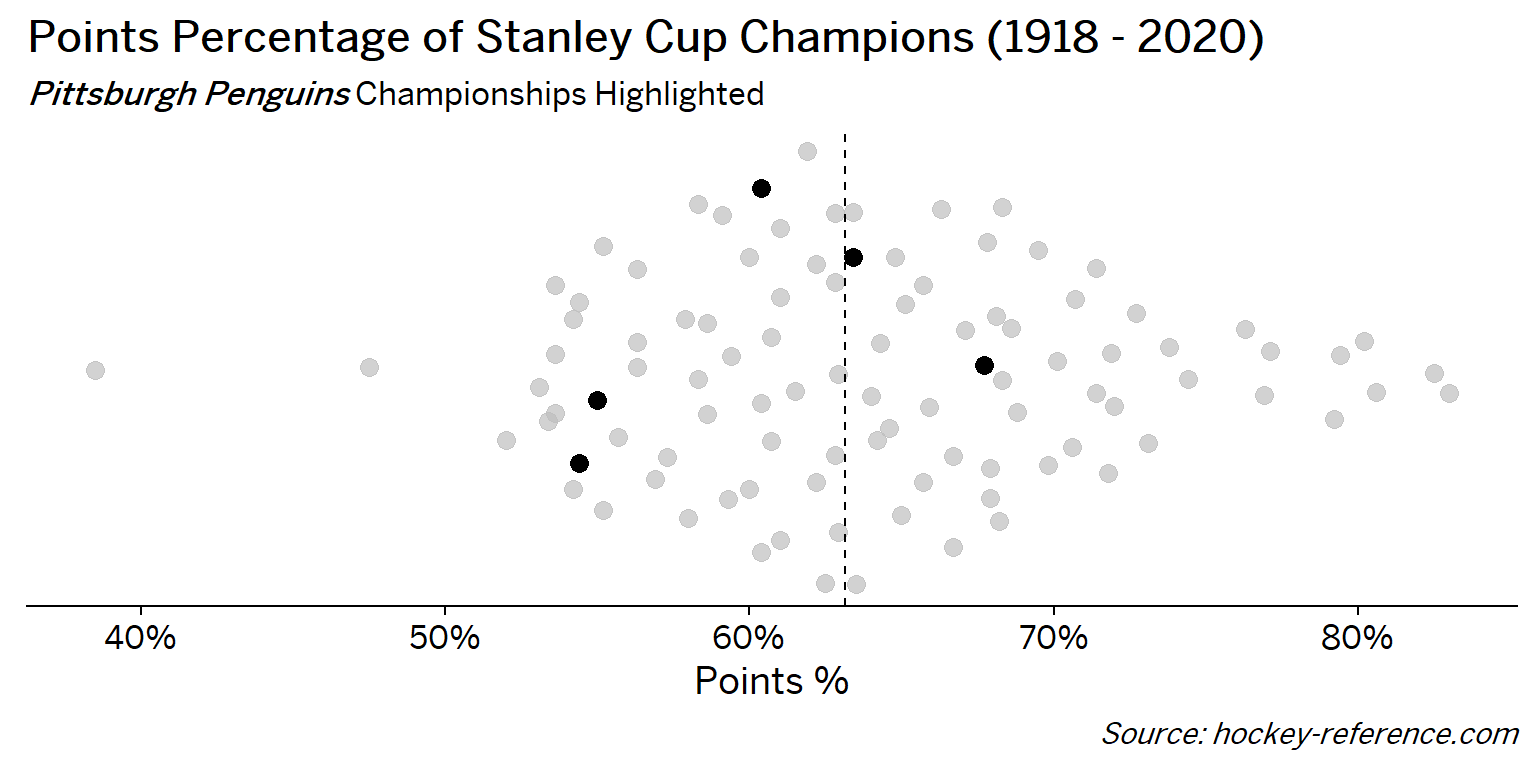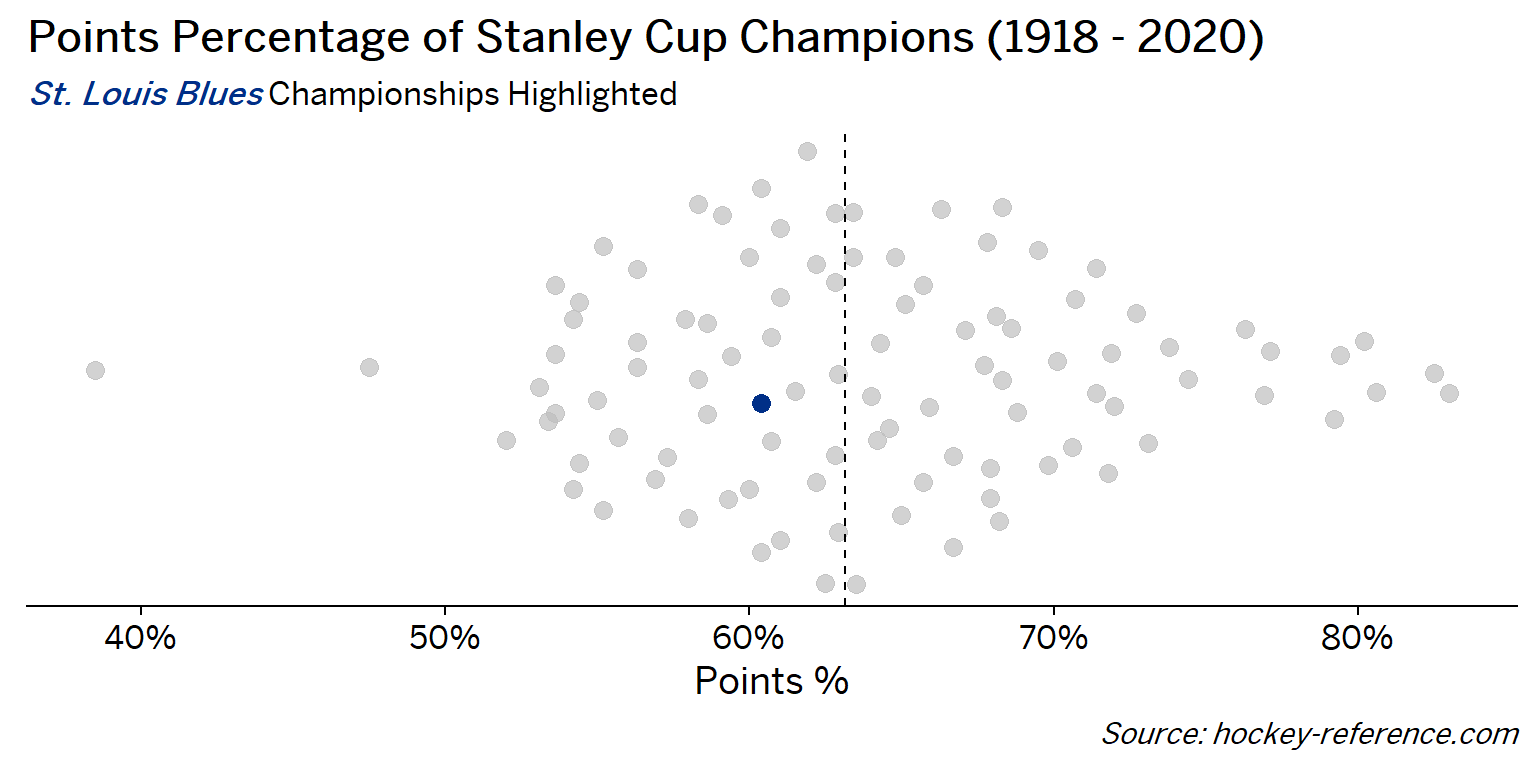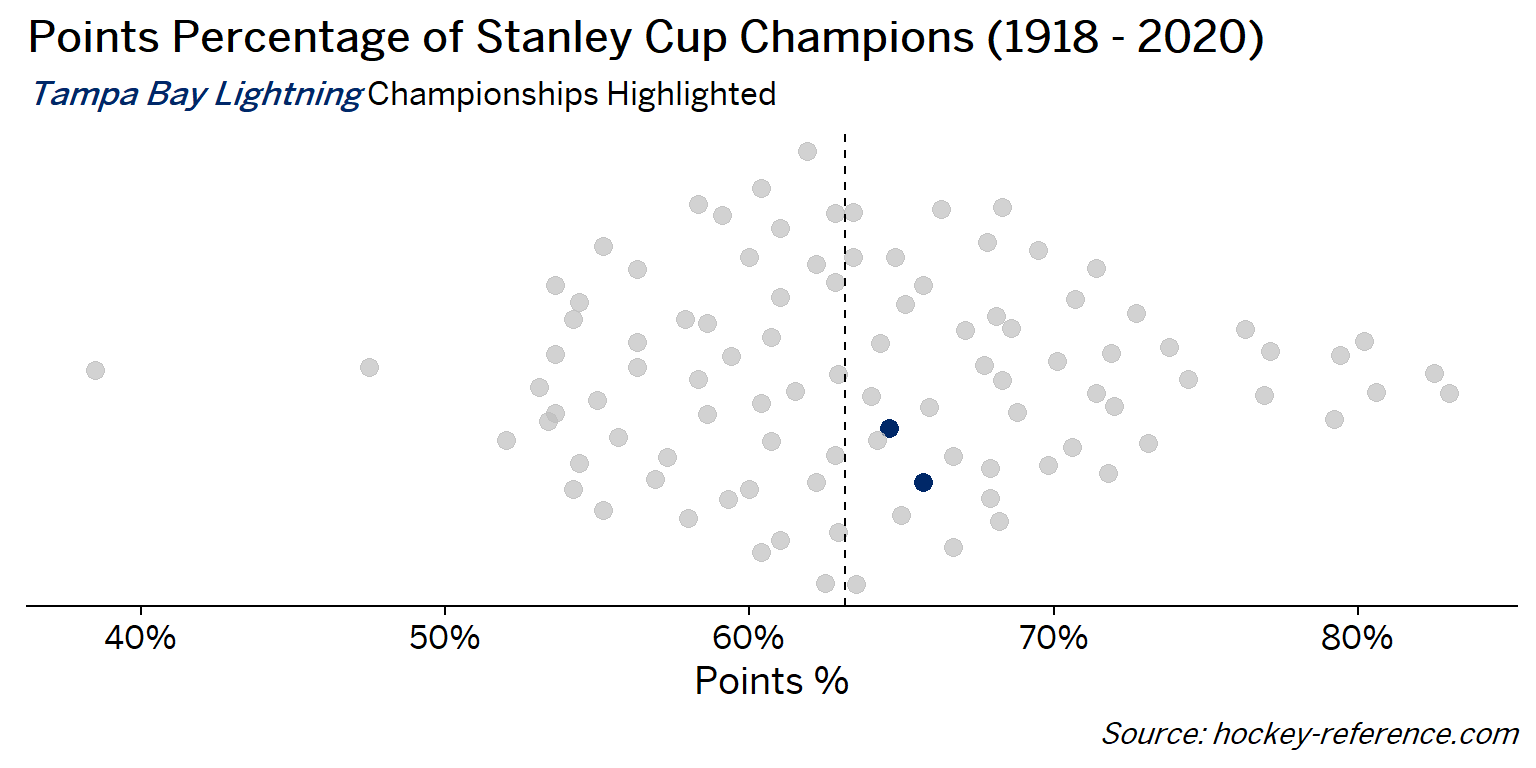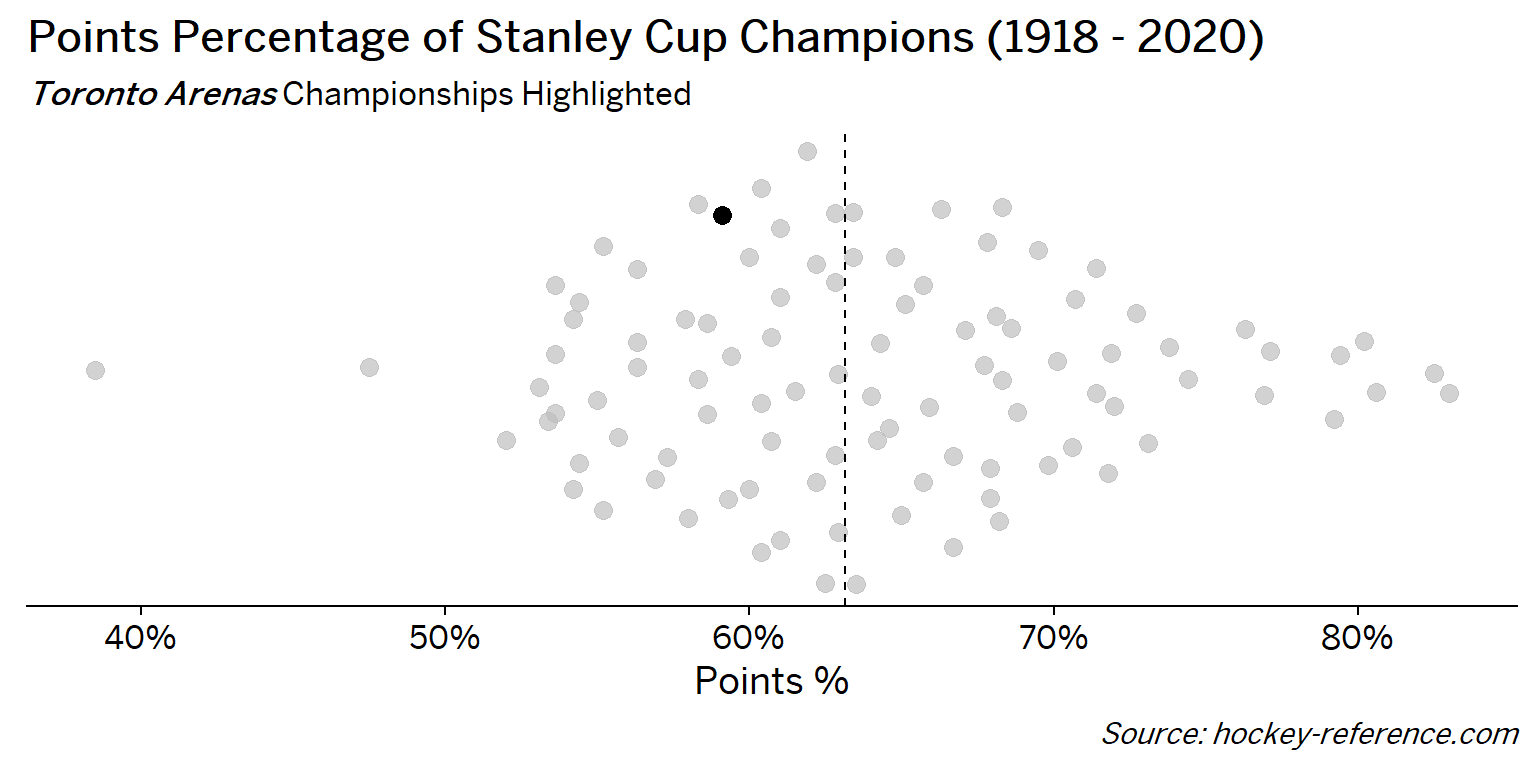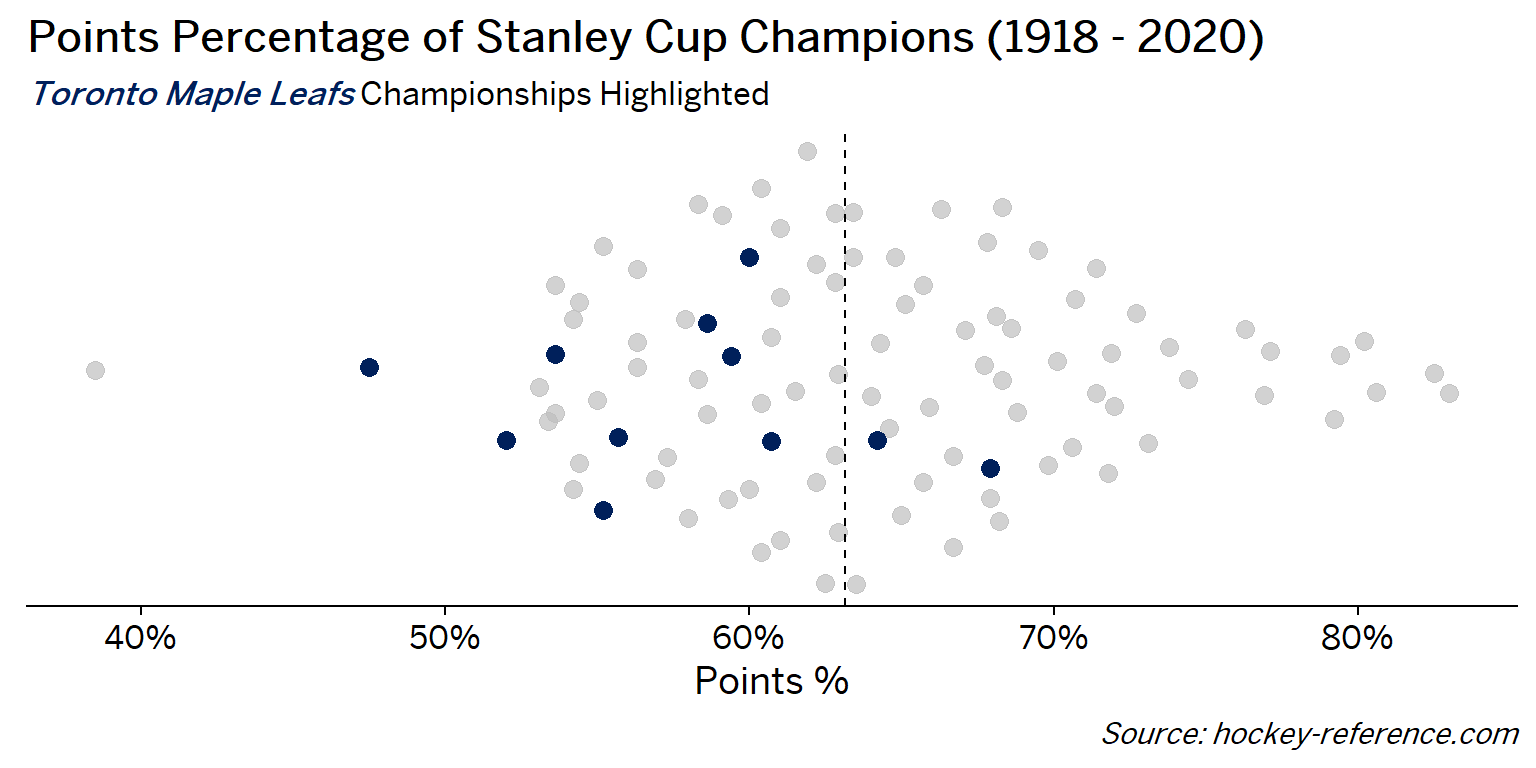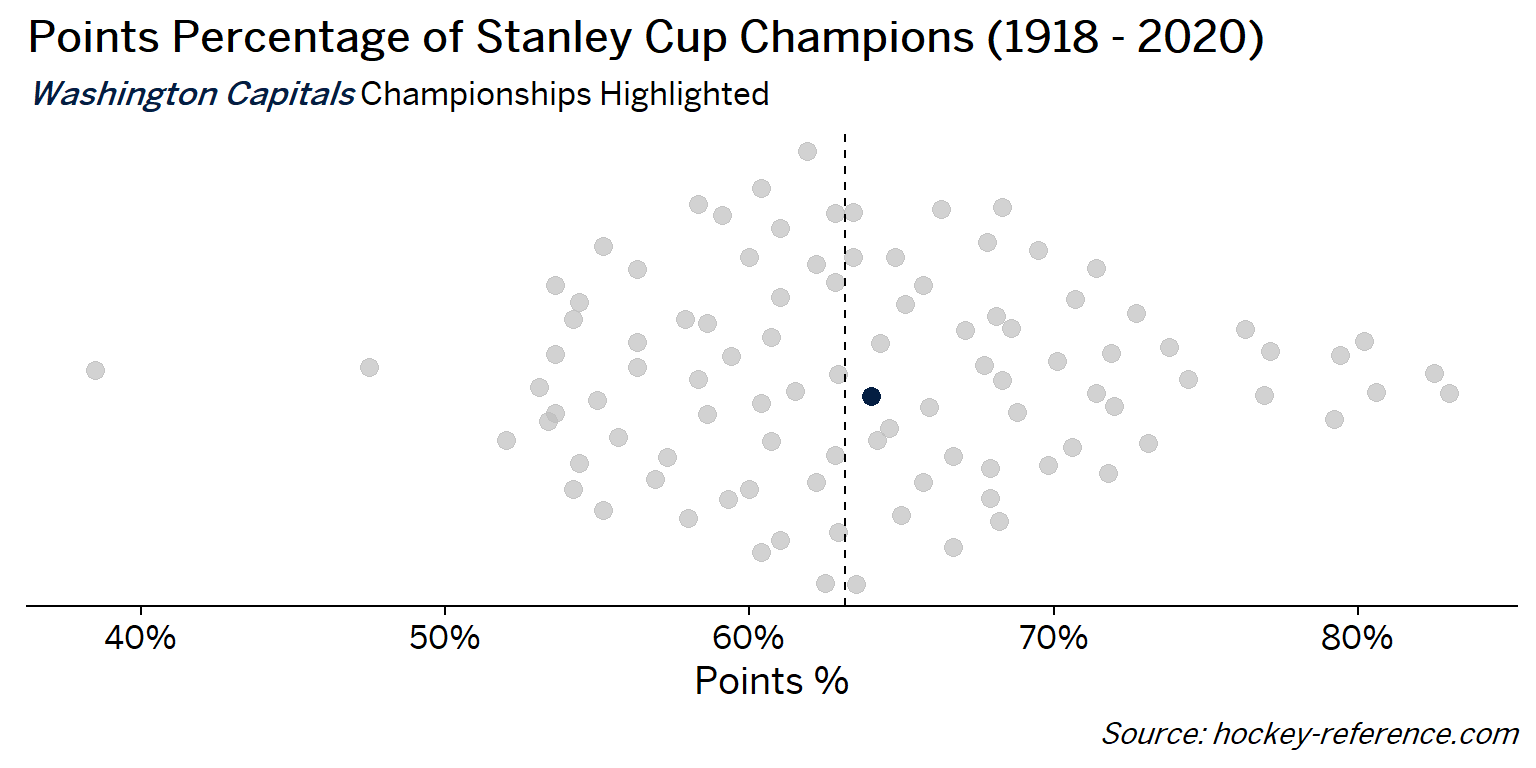Looking at the results of this plot we see that the Montreal Canadiens have been the most frequent winner as well as the team that makes up most of those 80%+ seasons. On the other hand, the Chicago Blackhawks have the honor of being the overachieving team that won despite having a sub-40% points percentage.

### Making a Histogram with Team Logos

An alternative view to the one above that doesn’t require highlighting would be to make a conventional histogram but using the team icons rather than points or bars. The `ggimage` package allows for a `geom_image` to be used by referencing a URL for an image. Fortunately the `teamcolors` package contains a dataset with links to logos for current NHL team. However, for some of the champion teams that no longer exist I needed to manually add their logos.

In this code block I manually create bin widths of 2.5% using the floor trick mentioned above and make use to a dummy variable to create the stacking effect for each of the logos. Then the `geom_image` references the URLs contained in the ‘logo’ column.

``````nhl_w_color %>%
left_join(teamcolors::teamcolors %>% select(name, logo),
by = c('Team' = 'name')) %>%
mutate(
logo = case_when(
Team == 'Montreal Maroons' ~ 'https://content.sportslogos.net/logos/1/40/thumbs/4039161926.gif',
Team == 'Toronto Arenas' ~ 'https://content.sportslogos.net/logos/1/996/thumbs/lgtkven0lgs74prrf26p6rmes.gif',
Team == 'Toronto St. Patricks' ~ 'https://content.sportslogos.net/logos/1/997/thumbs/6438.gif',
TRUE ~ logo
),
point_pct_bckt = floor(pts_pct*100/2.5)*2.5/100
) %>%
arrange(point_pct_bckt, desc(Team)) %>%
group_by(point_pct_bckt) %>%
mutate(
dummy = 1,
y_val = (cumsum(dummy)-1)*3
) %>%
ggplot(aes(x = point_pct_bckt, y = y_val)) +
geom_image(aes(image = logo),
asp = 1.5,
size = .05
) +
geom_vline(xintercept = quantile(nhl_data\$pts_pct, .5), lty = 2) +
labs(x = "Points %", y = "",
title = "Points Percentage of Stanley Cup Champions (1918 - 2020)",
caption = "*Source: hockey-reference.com*") +
scale_x_continuous(labels = scales::percent_format(accuracy =)) +
cowplot::theme_cowplot() +
theme(
text = element_text( family = 'BentonSans Regular'),
axis.title.y = element_blank(),
axis.text.y = element_blank(),
axis.ticks.y = element_blank(),
axis.line.y = element_blank(),
plot.caption = element_markdown(),
plot.subtitle = element_markdown(),
plot.margin = unit(rep(1.2, 4), "cm"),
plot.title = element_text(hjust = .7)
)``````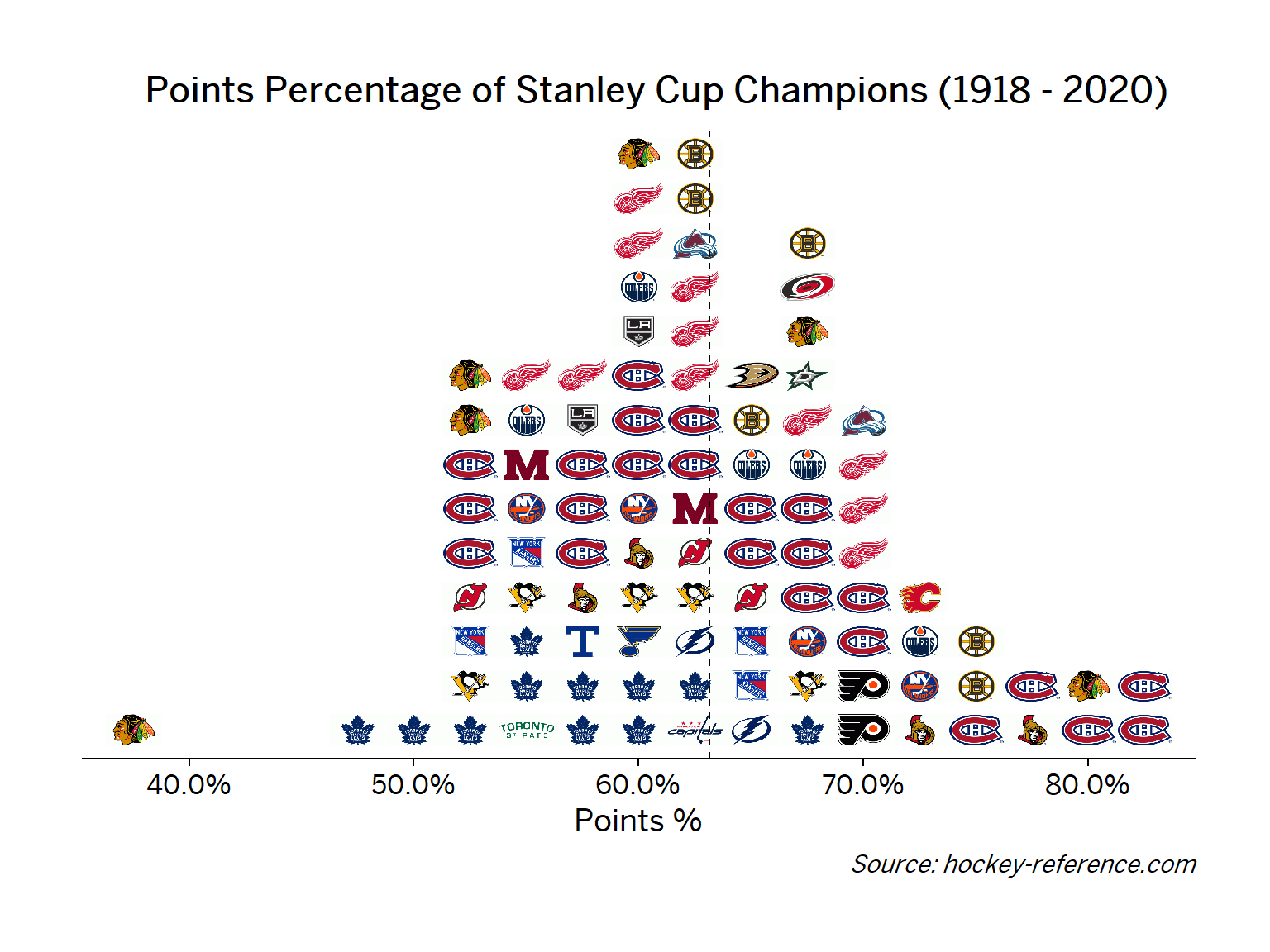Now its much easier to see that Montreal makes up most of the dominant teams while Chicago has been both dominant and on the lower ends of the distribution.

## Concluding Thoughts

The `ggplot2` ecosystem is quite impressive and this post hardly scratches the surface of all the possible options. However, in this post I show 4 ways a single variable, points percentage of NHL Stanley Cup Champions, can be represented.

• First, `geom_density` creates a baseline distribution
• `geom_density_ridge` from `ggridges` can stratify that initial density plot over another variable
• `geom_quasirandom` from `ggbeeswarm` will make a ‘violin-type’ plot but with specific points that can then be operated on.
• Finally, `ggimage` can change the geom to reference image URLs.

And as a bonus, I dynamically generated the tabsets for all the teams!

## Appendix: Code for Dynamic Tab Generation in Blogdown

``````##Construct Tabs
cat('<ul class="nav nav-pills nav-fill"> \n')
for(t in sort(unique(nhl_data\$Team))){
tid = str_to_lower(str_remove_all(t, ' |\\.'))
cat(glue('<li class="nav-item"><a class = "nav-link {active}" data-toggle="tab" href="#{tid}">{t}</a></li> \n',
active = if_else(t == sort(unique(nhl_data\$Team)), "active", "")))
}
cat('</ul> \n')

cat('<div class="tab-content"> \n')

for(t in sort(unique(nhl_data\$Team))){
tid = str_to_lower(str_remove_all(t, ' |\\.'))
cat(glue('<div id="{tid}" class="tab-pane {active}"> \n',
active = if_else(t == sort(unique(nhl_data\$Team)), "show active", "")))
set.seed(20201121)

g <- nhl_w_color %>%
mutate(color = if_else(Team == glue('{t}'),
color,
alpha("grey", 0.7))) %>%
ggplot(aes(y = 1, x = pts_pct, color = color)) +
geom_quasirandom(method = "tukeyDense", groupOnX=F, size = 3, width = 0.2) +
geom_vline(xintercept = quantile(nhl_data\$pts_pct, .5), lty = 2) +
labs(x = "Points %", y = "",
title = "Points Percentage of Stanley Cup Champions (1918 - 2020)",
subtitle = glue("<span style='color:{col};'><b><i>{t}</i></b></span> Championships Highlighted",
col = nhl_w_color %>%
filter(Team == glue('{t}')) %>%
pull(color) %>%
unique
),
caption = "*Source: hockey-reference.com*") +
scale_color_identity(guide = F) +
scale_x_continuous(labels = scales::percent_format(accuracy = 1)) +
cowplot::theme_cowplot() +
theme(
text = element_text( family = 'BentonSans Regular'),
axis.title.y = element_blank(),
axis.text.y = element_blank(),
axis.ticks.y = element_blank(),
axis.line.y = element_blank(),
plot.caption = element_markdown(),
plot.subtitle = element_markdown(),
plot.margin = unit(rep(1.2, 4), "cm"),

)

print(g)

cat("</div> \n")
}
cat("</div> \n")``````